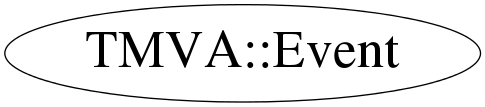# class TMVA::Event

## Function Members (Methods)

public:
 ~Event() static void ClearDynamicVariables() void CopyVarValues(const TMVA::Event& other) TMVA::Event Event() TMVA::Event Event(const TMVA::Event&) TMVA::Event Event(const vector*&, UInt_t nvar) TMVA::Event Event(const vector&, UInt_t theClass, Double_t weight = 1., Double_t boostweight = 1.) TMVA::Event Event(const vector& values, const vector& targetValues, UInt_t theClass = 0, Double_t weight = 1., Double_t boostweight = 1.) TMVA::Event Event(const vector& values, const vector& targetValues, const vector& spectatorValues, UInt_t theClass = 0, Double_t weight = 1., Double_t boostweight = 1.) Double_t GetBoostWeight() const UInt_t GetClass() const UInt_t GetNSpectators() const UInt_t GetNTargets() const UInt_t GetNVariables() const Double_t GetOriginalWeight() const Float_t GetSpectator(UInt_t ivar) const vector& GetSpectators() const vector& GetSpectators() const Float_t GetTarget(UInt_t itgt) const vector& GetTargets() const vector& GetTargets() const Float_t GetValue(UInt_t ivar) const vector& GetValues() const vector& GetValues() const Double_t GetWeight() const Bool_t IsDynamic() const TMVA::Event& operator=(const TMVA::Event&) void Print(ostream& o) const void ScaleBoostWeight(Double_t s) const void SetBoostWeight(Double_t w) const void SetClass(UInt_t t) void SetDoNotBoost() const static void SetIgnoreNegWeightsInTraining(Bool_t) static void SetIsTraining(Bool_t) void SetSpectator(UInt_t ivar, Float_t value) void SetTarget(UInt_t itgt, Float_t value) void SetVal(UInt_t ivar, Float_t val) void SetVariableArrangement(vector*const m) const void SetWeight(Double_t w)

## Data Members

private:
 Double_t fBoostWeight internal weight to be set by boosting algorithm UInt_t fClass class number Bool_t fDoNotBoost mark event as not to be boosted (used to compensate for events with negative event weights Bool_t fDynamic is set when the dynamic values are taken vector fSpectators "visisting" variables not used in MVAs ; mutable, to be able to copy the dynamic values in there vector fTargets target values for regression vector fValues the event values ; mutable, to be able to copy the dynamic values in there vector* fValuesDynamic the event values vector fValuesRearranged the event values ; mutable, to be able to copy the dynamic values in there vector* fVariableArrangement needed for MethodCategories, where we can train on other than the main variables Double_t fWeight event weight (product of global and individual weights) static Bool_t fgIgnoreNegWeightsInTraining static Bool_t fgIsTraining mark if we are in an actual training or "evaluation/testing" phase --> ignoreNegWeights only in actual training !

## Class Charts## Function documentation

Event()
``` copy constructor
```
Event(const vector<Float_t>& values, const vector<Float_t>& targetValues, UInt_t theClass = 0, Double_t weight = 1., Double_t boostweight = 1.)
``` constructor
```
Event(const vector<Float_t>& values, const vector<Float_t>& targetValues, const vector<Float_t>& spectatorValues, UInt_t theClass = 0, Double_t weight = 1., Double_t boostweight = 1.)
``` constructor
```
Event(const vector<Float_t>& , UInt_t theClass, Double_t weight = 1., Double_t boostweight = 1.)
``` constructor
```
Event(const vector<Float_t*>*& , UInt_t nvar)
``` constructor for single events
```
Event(const TMVA::Event& )
``` copy constructor
```
~Event()
``` Event destructor
```
void SetVariableArrangement(vector<UInt_t>*const m) const
``` set the variable arrangement
```
void CopyVarValues(const TMVA::Event& other)
``` copies only the variable values
```
Float_t GetValue(UInt_t ivar) const
``` return value of i'th variable
```
Float_t GetSpectator(UInt_t ivar) const
``` return spectator content
```
const std::vector<Float_t>& GetValues() const
``` return value vector
```
UInt_t GetNVariables() const
``` accessor to the number of variables
```
UInt_t GetNTargets() const
``` accessor to the number of targets
```
UInt_t GetNSpectators() const
``` accessor to the number of spectators
```
void SetVal(UInt_t ivar, Float_t val)
``` set variable ivar to val
```
void Print(ostream& o) const
``` print method
```
void SetTarget(UInt_t itgt, Float_t value)
``` set the target value (dimension itgt) to value
```
void SetSpectator(UInt_t ivar, Float_t value)
``` set spectator value (dimension ivar) to value
```
Double_t GetWeight() const
``` return the event weight - depending on whether the flag
*IgnoreNegWeightsInTraining* is or not. If it is set AND it is
used for training, then negetive event weights are set to zero !
NOTE! For events used in Testing, the ORIGINAL possibly negative
event weight is used  no matter what
```
void SetIsTraining(Bool_t )
``` when this static function is called, it sets the flag whether
events with negative event weight should be ignored in the
training, or not.
```

``` when this static function is called, it sets the flag whether
events with negative event weight should be ignored in the
training, or not.
```
Bool_t IsDynamic() const
``` accessors
```
`{return fDynamic; }`
Double_t GetOriginalWeight() const
`{ return fWeight; }`
Double_t GetBoostWeight() const
`{ return TMath::Max(Double_t(0.0001),fBoostWeight); }`
UInt_t GetClass() const
`{ return fClass; }`
std::vector<Float_t>& GetValues()
```For a detailed explanation, please see the heading "Avoid Duplication in const and Non-const Member Function," on p. 23, in Item 3 "Use const whenever possible," in Effective C++, 3d ed by Scott Meyers, ISBN-13: 9780321334879.
http://stackoverflow.com/questions/123758/how-do-i-remove-code-duplication-between-similar-const-and-non-const-member-func
```
Float_t GetTarget(UInt_t itgt) const
`{ return fTargets.at(itgt); }`
std::vector<Float_t>& GetTargets()
`{ return fTargets; }`
const std::vector<Float_t>& GetTargets() const
`{ return fTargets; }`
std::vector<Float_t>& GetSpectators()
`{ return fSpectators; }`
const std::vector<Float_t>& GetSpectators() const
`{ return fSpectators; }`
void SetWeight(Double_t w)
`{ fWeight=w; }`
void SetBoostWeight(Double_t w) const
`{ fDoNotBoost ? fDoNotBoost = kFALSE : fBoostWeight=w; }`
void ScaleBoostWeight(Double_t s) const
`{ fDoNotBoost ? fDoNotBoost = kFALSE : fBoostWeight *= s; }`
void SetClass(UInt_t t)
`{ fClass=t; }`
void SetDoNotBoost() const
`{ fDoNotBoost = kTRUE; }`
void ClearDynamicVariables()
`{}`